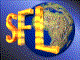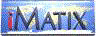| iMatix home page | << | < | > | >>SFLVersion 2.11

### sym_create_symbol_

```#include "sflsymb.h"
SYMBOL *
sym_create_symbol_ (
SYMTAB *table,                      /*  Symbol table to insert into      */
const char *name,                   /*  Name of symbol to create         */
const char *value,                  /*  Value of symbol to create        */
const char *filename,               /*  Name of source file making call  */
size_t      lineno)                 /*  Line number in calling source    */
```

#### Synopsis

Creates a new symbol in the specified table. Use the sym_create_symbol macro to call this function! Returns a SYMBOL pointer to the created symbol, or NULL if there was not enough memory to create the symbol. Initialises the symbol name and value to the values supplied. Sets symbol data to NULL. You can set this yourself if you need to, after calling this function. Use mem_alloc() or mem_strdup() to assign values to the data block, otherwise you may cause problems when you delete the symbol or symbol table, since these functions free these fields. You can create several symbols with the same name; the last-defined is always placed before older instances and will be found first by sym lookup symbol().

#### Examples

```    SYMTAB
*symbol_table;
SYMBOL
*new_symbol;

symbol_table = sym_create_table ();
ASSERT (symbol_table);
new_symbol = sym_create_symbol (symbol_table, "This name", "This value");
if (new_symbol)
{
new_symbol-> data = mem_alloc (sizeof (my_block));
memcpy (new_symbol-> data, my_block);
}
```

#### Source Code - (sflsymb.c)

```{
SYMBOL
*symbol;                        /*  Allocated symbol                 */
int
hash;                           /*  Hash bucket no. for symbol       */

ASSERT (table);

symbol = mem alloc  (NULL, sizeof (*symbol) + strlen (name) + 1,
filename, lineno);
if (symbol)
{
/*  Set the symbol pointers and fields                               */
hash = sym hash (name);
symbol-> next   = table-> symbols;
symbol-> prev   = NULL;
symbol-> h_next = table-> hash [hash];
symbol-> h_prev = NULL;
symbol-> name   = (char *) symbol + sizeof (*symbol);
symbol-> value  = mem_strdup (value);
symbol-> data   = NULL;
symbol-> hash   = (byte) hash;
strcpy (symbol-> name, name);

if (table-> symbols)
table-> symbols-> prev = symbol;
table-> symbols = symbol;

if (table-> hash [hash])
table-> hash [hash]-> h_prev = symbol;
table-> hash [hash] = symbol;
table-> size++;
}
return (symbol);
}
```

 | << | < | > | >>Copyright © 1996-2000 iMatix Corporation